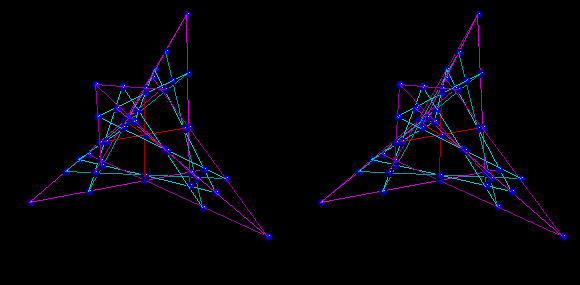# The generalized quadrangle of order (4,2)This animation will take a quite a while to load. It shows a spatial model of the generalized quadrangle of order (4,2). Embedded in this model is a model of the generalized quadrangle of order (2,2) and a Schlaefli double-six. The 15 lines in the generalized quadrangle of order (2,2) are the 12 purple and the 3 red lines. The lines of the double six are the remaining 12 lines in the model.